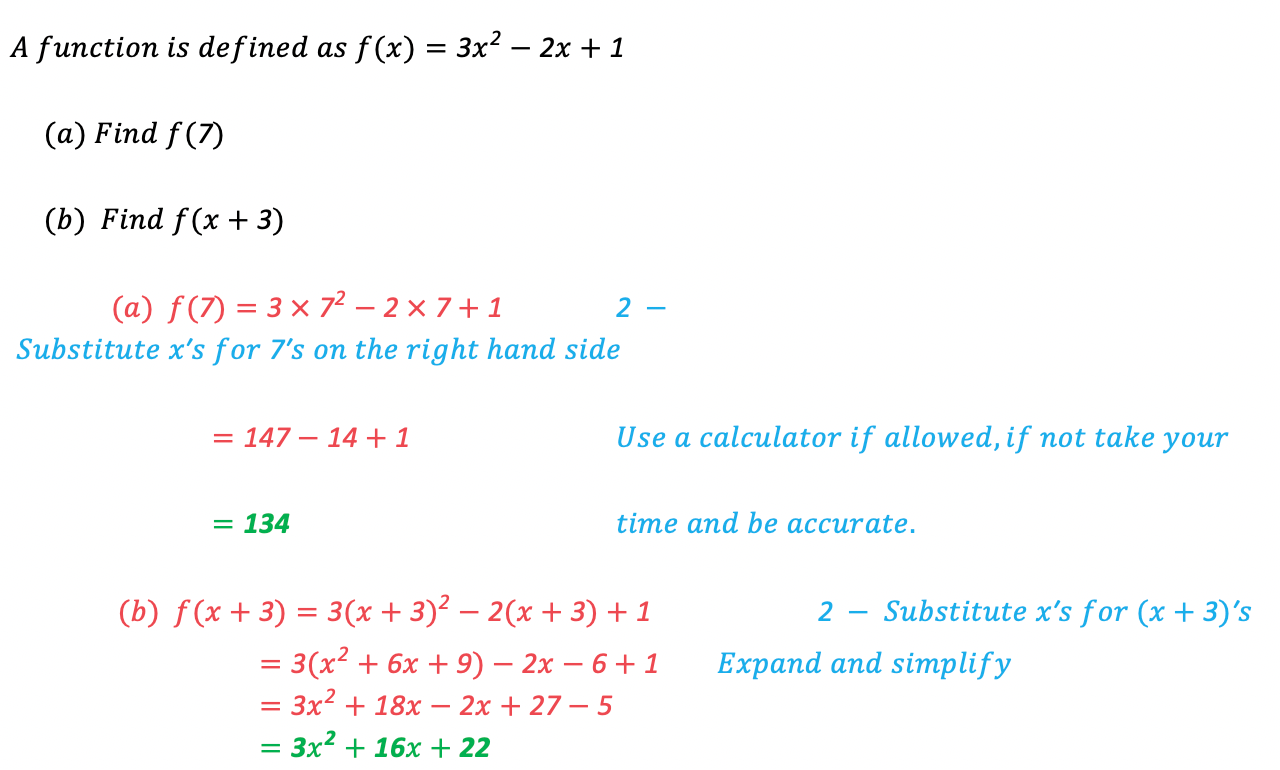# Edexcel IGCSE Maths 复习笔记 3.2.1 Functions - Basics

Edexcel IGCSE Maths 复习笔记 3.2.1 Functions - Basics

#### What is a function?

• A function is simply a mathematical “machine” that takes one set of numbers and changes them into another set of numbers according to a set ruleeg. If the function (rule) is “double the number and add 1”
• Putting 3 in to the function would give 2 × 3 + 1 = 7 out
• Putting -4 in would give 2 × (-4) + 1 = -7 out
• Putting a in would give 2a + 1 out

• The number being put into the function is often called the input
• The number coming out of the function is often called the output

#### What does a function look like?

• A function f can be written as:f(x) = … or f : x ↦ …which mean exactly the same thing
• eg. The function with the rule “triple the number and subtract 4” would be written:f(x) = 3x – 4 or f:x ↦ 3x – 4
• In such cases, x would be the input and f would be the output
• Sometimes functions don’t have names like f and can be written as y = …eg. y = 3x – 4

#### How does a function work?

1. A function has an INPUT (x) and OUTPUT (f or y)
2. Whatever goes in the bracket (instead of x)with f, replaces the x on the other sideeg. For the function f(x) = 2x + 1
• f(3) = 2 × 3 + 1=7
• f(-4) = 2 × (-4) + 1 = -7
• f(a) = 2a + 1

#### Worked Example